## Buoyancy Factor with Two Different Fluid Weights in The WellBuoyancy Factor is the factor that is used to compensate loss of weight due to immersion in drilling fluid and you can find more information from this article > buoyancy factor calculation .  In that article, it demonstrates the buoyancy formula only for one fluid in the wellbore. However, this time, we will have the details about buoyancy factor when inside and outside fluid are different.

Buoyancy factor with different fluid inside and outside of tubular is listed below;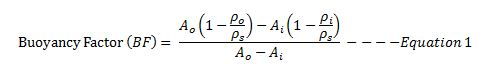Where;

Ao is an external area of the component.

Ai is an internal area of the component.

ρo is fluid density in the annulus at the component depth in the wellbore.

ρi is fluid density in the component depth in the wellbore.

ρs is steel weight density. Steel density is 65.4 ppg.

If you can the same mud weight inside and outside, the equation 1 will be like this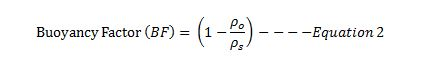Let’s take a look at the following example to get more understanding.

Example

13-3/8” casing shoe was at 2,500’MD/2,000’TVD

9-5/8” casing was run to 6,800’MD/6,000 TVD.

9-5/8” casing weight is 40 ppf and casing ID is 8.835 inch.

Current mud weight is 9.5 ppg oil based mud.

The well bore diagram is show below (Figure 1).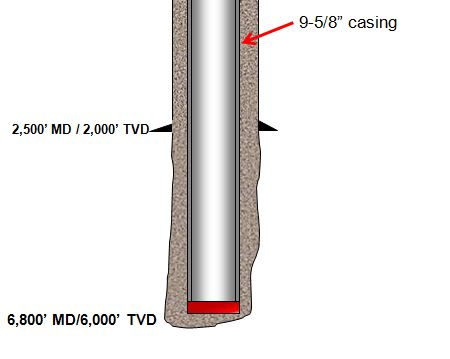Figure 1 – Wellbore Diagram

The well is planned to cement from shoe to surface and the planned cement weight is 14.0 ppg. The displacement fluid is drilling mud currently used.

• Air weight of casing string
• Buoyed weight of casing in drilling mud
• Buoyed weight of casing when cement is inside casing and drilling mud is outside casing
• Buoyed weight of casing when cement is outside casing and drilling mud is inside casing

Air weight of casing string

Air weight of casing string, lb = length of casing, ft × casing weight, lb/ft

Air weight of casing string, lb = 6,800 × 40 = 272,000 lb

Buoyed weight of casing in drilling mudFigure 2 – Buoyed Weight When Submersed In Drilling Mud

Buoyed weight = Buoyancy Factor (BF) × Air Weight of Casing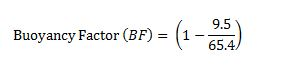Buoyancy Factor (BF) = 0.855

Buoyed weight = 0.855 × 272,000 = 232,489 lb

Buoyed weight of casing when cement is inside casing and drilling mud is outside casing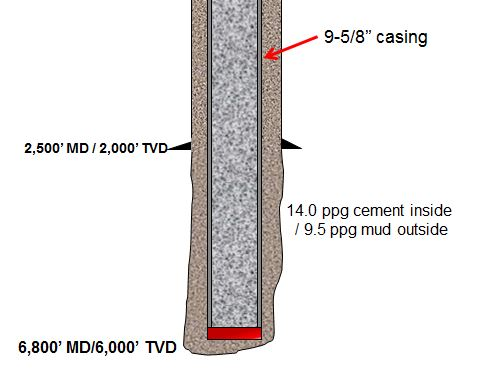Figure 3 – Buoyed weight of casing when cement is inside casing and drilling mud is outside casing

We will apply the Equation-1 for this case.

Ao is an external area of the component.

Ao = π × (Outside Diameter of casing)2 ÷ 4

Ao = π × (9.625)2 ÷ 4 = 72.76 square inch

Ai is an internal area of the component.

Ai = π × (Inside Diameter of casing)2 ÷ 4

Ai = π × (8.835)2 ÷ 4 = 61.31 square inch

ρo = 9.5 ppf (mud in the annulus)

ρi = 14.0 ppg (cement inside casing)

ρs = 65.4 ppg.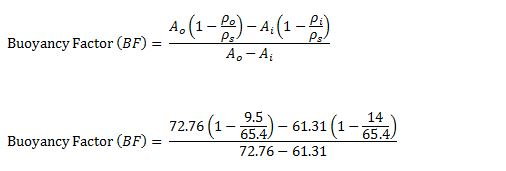Buoyancy Factor (BF) = 1.22

Buoyed weight = 1.22 × 272,000 = 331,840 lb

Buoyed weight of casing when cement is outside casing and drilling mud is inside casing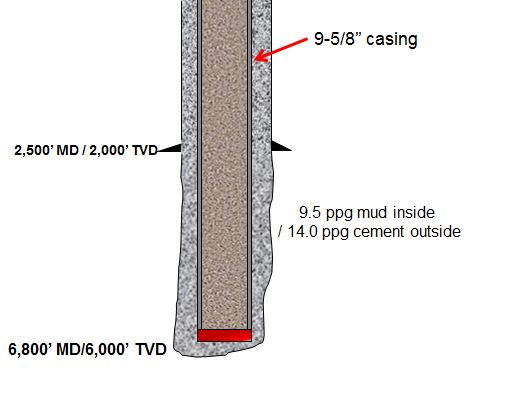Figure 4 – Buoyed weight of casing when cement is outside casing and drilling mud is inside casing

We will apply Equation-1 for this case as well.

All the calculation parameters are the same.

Ao = π × (9.625)2 ÷ 4 = 72.76 square inch

Ai = π × (8.835)2 ÷ 4 = 61.31 square inch

ρo = 9.5 ppg (mud in the annulus)

ρi = 14.0 ppg (cement inside casing)

ρs = 65.4 ppg.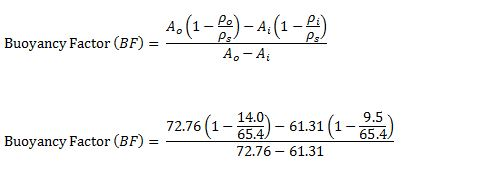Buoyancy Factor (BF) = 0.42

Buoyed weight = 0.42 × 272,000 = 114,240 lb

Conclusion: At different stage of the well, you may have different buoyed weight depending on density of fluid inside and outside of the component and it is not always that buoyed weight is less than air weight.

Reference book =>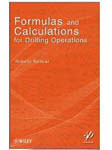Formulas and Calculations for Drilling Operations

Buoyancy factor is the factor that is used to compensate loss of weight due to immersion in drilling fluid and you can find the calculation from here https://www.drillingformulas.com/buoyancy-factor-calculation/.We have created a simple table to help people determine the buoyancy factor quickly. Let’s take a look at the table. In Figure 1, it shows the main page and you can select the mud weight range from 4.0 ppg to 19.0 ppg.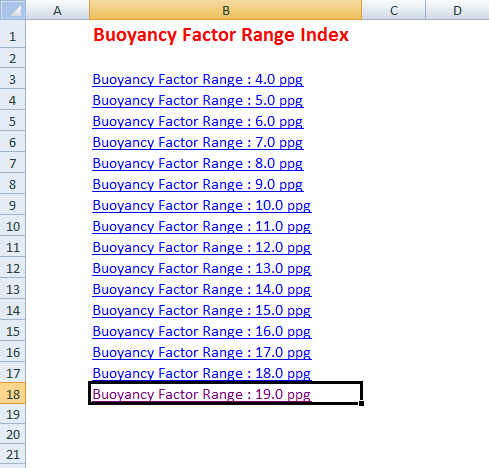Figure 1– Main Page BF Table

For instant, we choose 8.0 ppg and the table will show buoyancy from 8.0 – 8.9 ppg (Figure 2)Figure 2 – Buoyancy Factor for 8 ppg Range

## Buoyancy Factor CalculationBuoyancy Factor is the factor that is used to compensate loss of weight due to immersion in drilling fluid.

Before explaining any further, we will explain you about the basic concept of Buoyancy. Buoyancy is the upward force that keeps things afloat. The net upward buoyancy force equals to the amount of the weight of fluid displaced by the body volume. This force will make objects lighter when it immerses in fluid. For example, we feel ourselves lighter when we are in swimming pool because this is the effect of buoyancy.In drilling operation, we need to know how much weight of string of drill pipe, completion string, etc in drilling fluid. Therefore,  Buoyancy Factor is value that we need to know and be able to calculate this value. Please follow the formulas below to calculate Buoyancy Factor in different mud weight units, ppg and lb/ft3.

## Buoyancy Factor using mud weight in ppg

Buoyancy Factor (BF) = (65.5 – mud weight in ppg) ÷65.5

Note: 65.5 ppg is density of steel.

Example: Determine the buoyancy factor for a 13.0 ppg fluid:
BF = (65.5 – 13.0) ÷ 65.5
BF = 0.8015

## Buoyancy Factor using mud weight in  lb/ft3

Buoyancy Factor (BF) = (489 – mud weight in lb/ft3) ÷489

Note: 489 lb/ft3 is density of steel.

Example: Determine the buoyancy factor for a 100 lb/ft3 fluid:
BF = (489 – 100) ÷489
BF = 0.7955

## Buoyancy Factor using mud weight in kg/l

Buoyancy Factor (BF) = (7.85 – mud weight in kg/l) ÷7.85

Note: 7.85 kg/l is density of steel.

Example: Determine the buoyancy factor for a 1.1 kg/l fluid:
BF = (7.85 – 1.1) ÷7.85
BF = 0.860

## Buoyancy Factor Table

This table demonstrates buoyancy factor at different mud density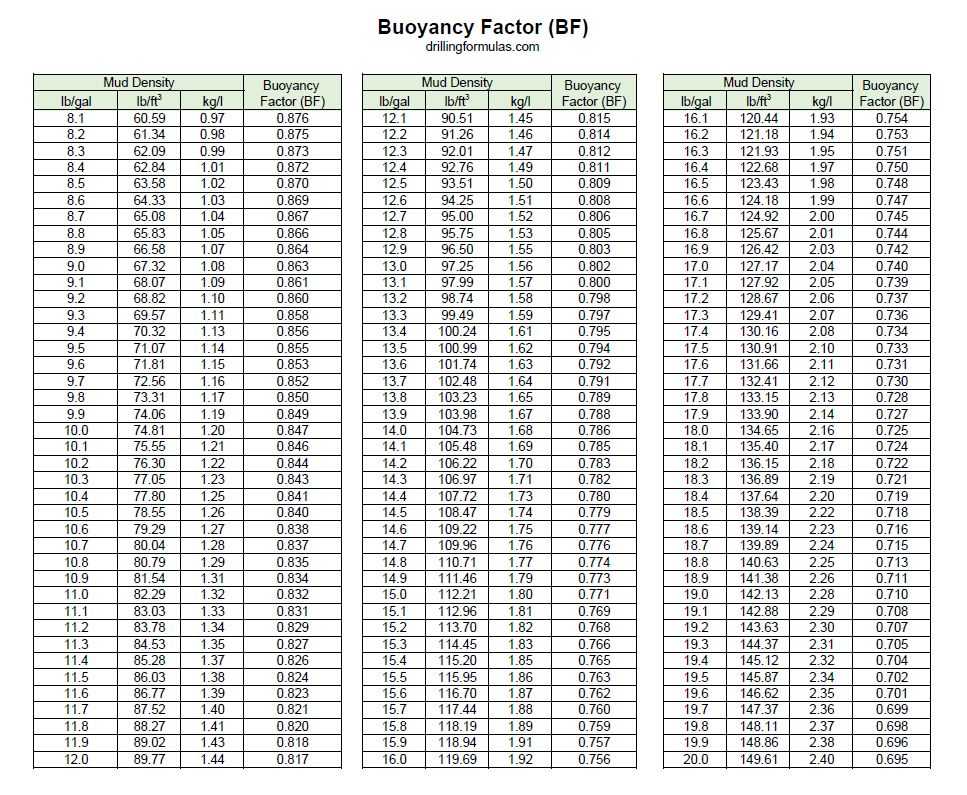Buoyancy Factor Table

## How to use the Buoyancy Factor to determine buoyed weight

In order to figure out the actual weight of drilling string in fluid, the air weight of drilling string times the buoyancy factor equal to actual weight in mud, called buoyed weight.

Buoyed weight of drill string = String weight in the air × Buoyancy Factor

Example: Determine the string weight in 13.0 ppg mud. Air weight of string is 350 klb.

The buoyancy factor for a 13.0 ppg fluid:
BF = (65.5 – 13.0) ÷ 65.5
BF = 0.8015

Buoyed weight of drill string = String weight in the air × Buoyancy Factor

The buoyed weight of drill string in 13.0 ppg mud = 350 x 0.8015 = 280.5 Klb.